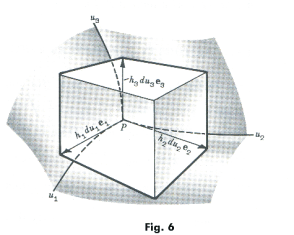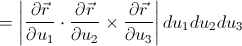## Pages

### 4. Volume element

We have just seen the differential form of the arc length {note. 3}, what about the volume element?

Let us consider the following figure,We know that from the vector analysis that the scalar triple product of the three basis vector gives the volume enclosed. The same trick is followed in finding the differential volume element of the curvilinear system of coordinate.

In the above figure, the three vector that we are concerned is the tangential vector of the curvilinear system $(u_{1},u_{2},u_{3})$.

Now, we know that,
Where, $(p,q = 1,2,3)$

We have, the differential volume element given as,..$1$
Our aim is now to find the value of the determinant in the above equation. There are so many question we can raise. One of the important one is, Is this determinant connected to the metric tensor of the reference curvilinear system? Our intuition can force us to say yes, but it's very difficult to grasp the relation in a single statement without some mathematical rigor. So, we do have to use certain trick of the matrix rules in order to come up with certain understanding.

First of all get familiar with the following determinant properties . {Specially property 8}

let us consider finding the following,
The square of the above simply implies this,

The result we got here is the answer for the square of the determinant. What we need is the value of the determinant only. So, when we square root both sides, we get the square root of the $g$ on the right hand side﻿. Thus, substituting the value of this determinant in $..1$ we get the desired result i.e.,

We have thus found the differential element of the volume.

(source:schaum outlines)